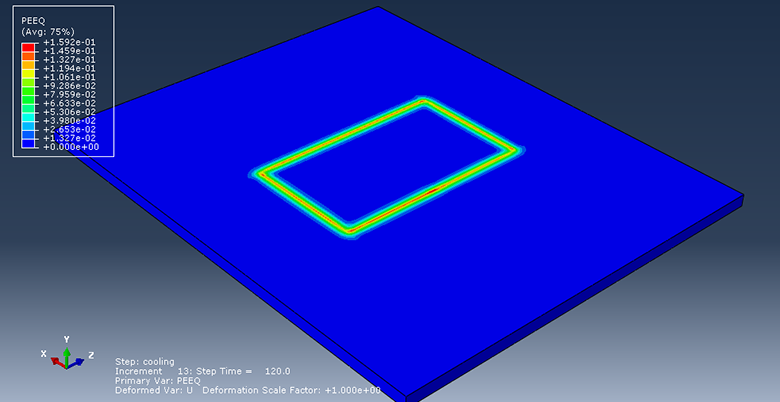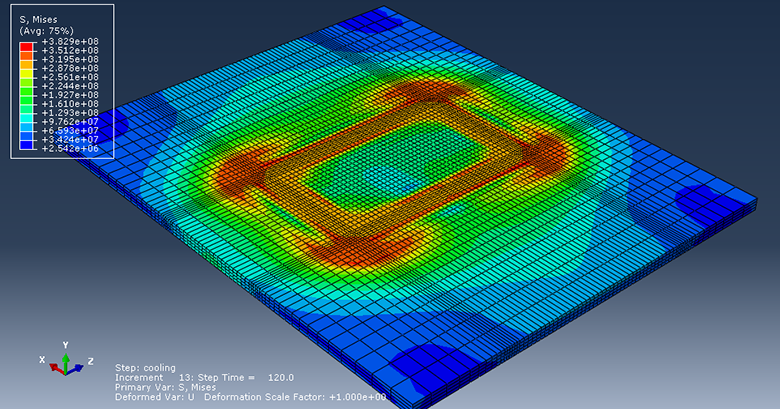پکیج آموزش پیشرفته آباکوس
پکیج جامع آباکوس ویژه رشته مکانیک
پکیج جامع آباکوس ژئوتکنیک و عمران

Simulation the Arc welding in a rectangular path using DFLUX subroutine Abaqus

In this example, we intend to simulate the Arc welding in a rectangular path using Dflux subroutine in the Abaqus software

We use volumetric heat flux for simulation of the welding process, and we use Goldak’s double-ellipsoid model for define volumetric heat flux, and we use the Dflux subroutine for define Goldak’s double-ellipsoid model

Dflux subroutine is used to define nonuniform heat flux

The welding torch moves through AB , BC , CD , DE and EA respectively , in fact welding Torch moves in a rectangular path

This problem is done in two steps

In step1 , we simulate welding process with duration of 32 seconds

In step2 , we simulate cooling process with duration of 120 seconds

To do so , we define two Coupled temp-displacement stepyou see equivalent plastic strain (PEEQ)

The material of the plate is SUS304

Density, thermal conductivity, specific heat, thermal expansion, elastic properties and plastic properties depend on the temperature

In this example , welding voltage is 22 volt , welding current is 110 ampere , efficiency is 0.8 , welding speed is 10mm/s

Goldak’s double-ellipsoid heat source model is adopted to calculate volumetric heat flux distributions as heat input around the welding pool

Equations of the Goldak’s heat source model is defined as a function of position and time together with a number of parameters

The constants a,b,Cf,Cr as shown in picture are heat source parameters that define the size and shape of the ellipses and these parameters are obtained from experimental analysisResidual Stress

Duration :29 minutes

Download link : full video , inp file , cae file , subroutine file, excel file and pdf file

Payment Method : Paypal

To purchase this tutorial , please contact us

Email : saeedofmoeini@gmail.com